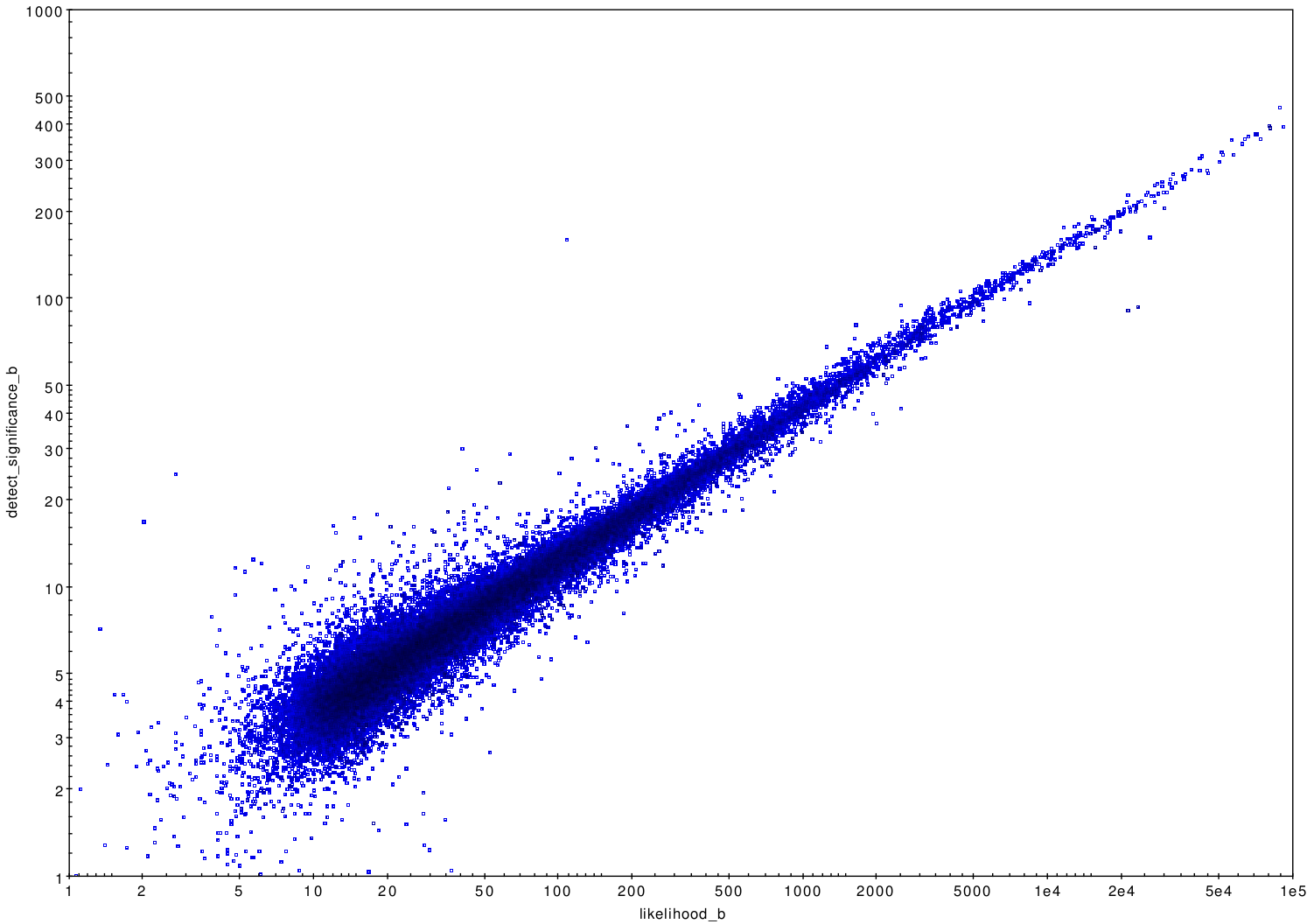Source Significance

In the first release of the CSC, detect and flux significance were related, since sources were accepted for inclusion in the catalog if their flux significance exceeded a given threshold.

In CSC 2.0, three separate metrics are used to describe the significance of detection and flux determination.

Detection Significance & Likelihood

The fundamental metric used to decide whether a source is included in CSC 2.0 is the likelihood,

where P is the probability that an MLE fit to a point or extended source model, in a region with no source, would yield a change in fit statistic as large or larger than that observed, when compared to a fit to background only.

The likelihood is closely related to the probability, Ppois, that a Poisson distribution with a mean background in the source aperture would produce at least the number of counts observed in the aperture. This quantity, called detect_significance, is also reported in CSC 2.0. Smoothed background maps are used to estimate mean background, and detect_significance is expressed in terms of the number of σ, z, in a zero-mean, unit standard deviation Gaussian distribution that would yield an upper integral probability Pgaus, from z to ∞, equivalent to Ppois. That is,

where

detect_significance vs. likelihooddetect_significance vs. likelihood

A plot of the detection significance vs. likelihood is shown in this figure.

Flux Significance

Flux significance is a simple estimate of the ratio of the flux measurement to its average error. The mode of the marginalized probability distribution for photflux_aper is used as the flux measurement and the average error, σe, is defined to be:

which are both used to estimate flux significance.

Table Columns

Master Sources Table:
significance, likelihood

The maximum likelihood and flux significance across all stacked observations and energy bands are reported as the master source significance and likelihood.

Stacked Observation Sources Table:
flux_significance, detect_significance, likelihood

Likelihood, detect significance, and flux significance are reported per band for all sources detected in the valid stack. The likelihood reported is the maximum of the likelihood determined from the MLE fit to all valid stack data, and the likelihoods from each individual observation, per band.

Per-Observation Sources Table:
flux_significance, likelihood

Likelihood and flux significance are reported per band for all detected sources that fall in the valid field of view. Likelihoods are computed for each source detection in a stack, from MLE fits to data from all valid observations for the source. Likelihoods from each individual observation are also computed.